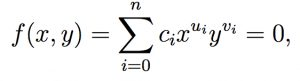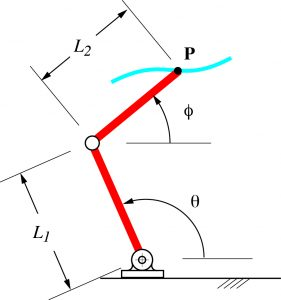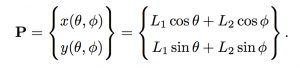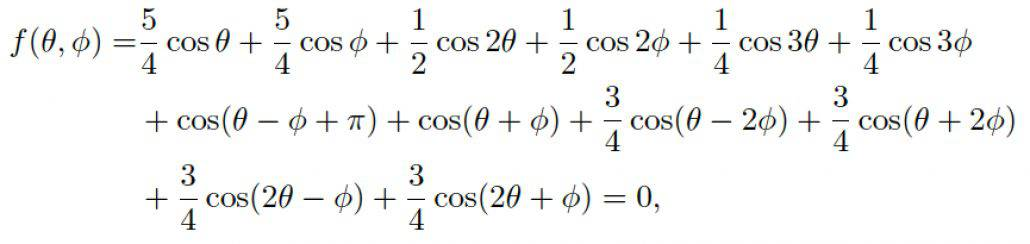## Mechanical computation and algebraic curves

A mechanical computer that draws an algebraic curve is a useful device, and  Mathematicians Michael Kapovich and John Millson have shown that a design always exists.

This is Yang Liu’s design of a mechanical system that draws an elliptic cubic curve.

Here is how it is done.

Algebraic curve. An algebraic curve is the set of points P=(x, y) with coordinates that satisfy an algebraic equation,Algebraic curve

where ui and vi are integer exponents, and ci are real coefficients.

Forward kinematics. In order to draw this curve, we use an RR serial chain shown in Figure 1.  All that is needed is to coordinate the angles of this chain so its end-point P traces the curve.Figure 1. The joints of an RR serial chain are coordinated to guide the point P along a specified curve.

The relationship between the joint angles and the coordinates P=(x, y) is given by the forward kinematics equations of this RR chain,RR forward kinematics

Kempe’s formula. In his 1876 paper, “On a general method of describing plane curvesof nth degree by linkwork,” A. B. Kempe substituted this into the equation of the curve and simplified the result to obtain,Kempe formula

Kempe interpreted this formula to be the x-coordinate of an N link serial chain, where each link has the length Aj and is positioned at the angle,The end-point of Kempe’s serial chain maintains the value x=C, which means it only moves along the y-direction.

Mechanical computation. Now connect cable drives to the two joints of the RR chain so they can be used as inputs to N mechanical computers that calculate the Psij angular values.  The output of the mechanical computers are connected by cable drives to the joints of Kempe’s serial chain.

The operations needed in the mechanical computers are simply multiplication and addition.  Multiplication of an angle by a given factor is achieved by using a cable drive with the ratio of the pulley sizes set to the specified factor.  The sum of two angular values is achieved using a bevel gear differential.

The result is a mechanical system that constrains the angles of an RR chain so it draws the specified algebraic curve within the workspace of RR serial chain.

Elliptic cubic curve. As an example, consider the elliptic cubic curve,Elliptic cubic curve

Let the RR chain have link lengths L1=L2=1, and obtain Kempe’s formula for this curve,Elliptic cubic formula

This equation has 12 terms, so Kempe’s serial chain has 12 links. The 12 mechanical computers connecting the angles of Kempes serial chain to the driving joints for the RR chain, include six additions and eight multiplications, Figure 2.Figure 2. The Kempe’s serial chain and the mechanical computations that constrain the RR serial chain to draw an elliptic cubic curve.

Rather than use differentials and cable drives, Kempe used linkages to perform the mechanical computations and couple the results. The outcome is a lot of links as can be seen in this animation provided by Alex Kobel.